Sorry, your browser does not support JavaScript!Home >

# Simulator introduction

Repository: IAMMETER-simulator

Welcome to let us know your thoughts here Integrate a virtual 3-phase energy meter (open source) into HA,use it to optimize your solar pv system

# Preface

The idea that let us start to develop this simulator comes from this post https://imeter.club/topic/320

One of our customers tries to optimize the use of solar excess output by the home assistant. Use IAMMETER`s energy meter to monitor the solar excess output and control the Tesla charge by the Home Assistant (use the excess solar as much as possible) .

we also want to participate in this project, to optimize the control logic together. But we do not have the Tesla and inverter in our office now. So we had an idea, develop a simulator to achieve such jobs.

Through this simulator, you can get a simulated WEM3080T.

Phase A is the measurement result of the solar inverter output(This result can be completely simulated by the simulator or get from a real energy meter).

The load profile is configured in the simulator, there are different load models (fixed,timer, configurable).

Then the simulator calculates the grid consumption automatically by the solar measurement result (simulated or real) and load(simulated) profile, and outputs the result in phase B.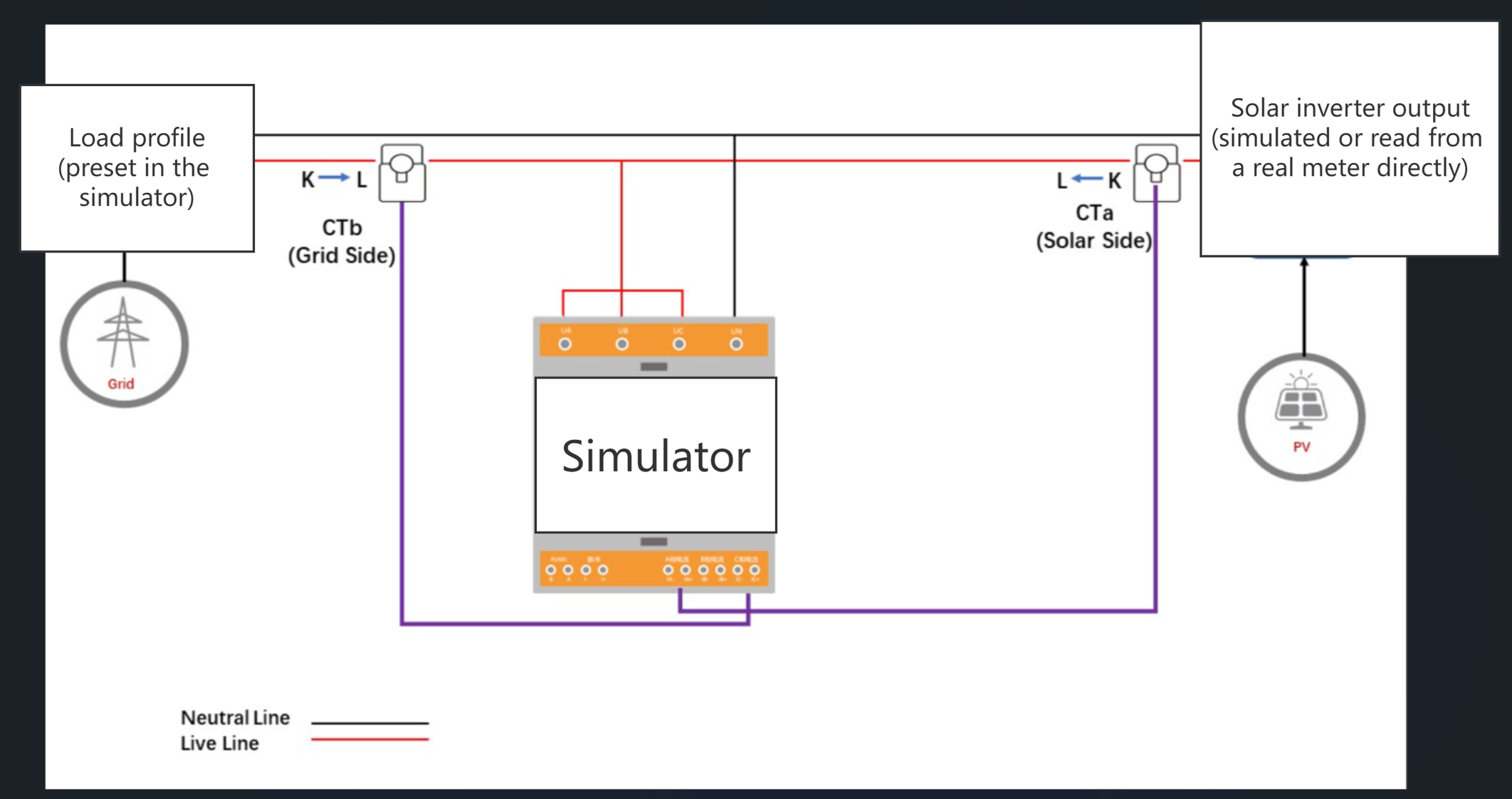# High light features

You can do such interesting jobs by this simulator.

• Optimize your load control strategy: create a load profile, change the control logic of the load and try to find out which logic can be achieved the maximum return from your solar PV system( To Improve the self use rate in your solar PV system).
• Forecast the solar PV revenue before the installation: simulated a solar PV output, compute the possible solar PV saving and income by the simulated solar data and your real electrical energy consumption data.

The simulated 3 phase energy meter can also be added into the Home assistant or IAMMETER, like the real hardware.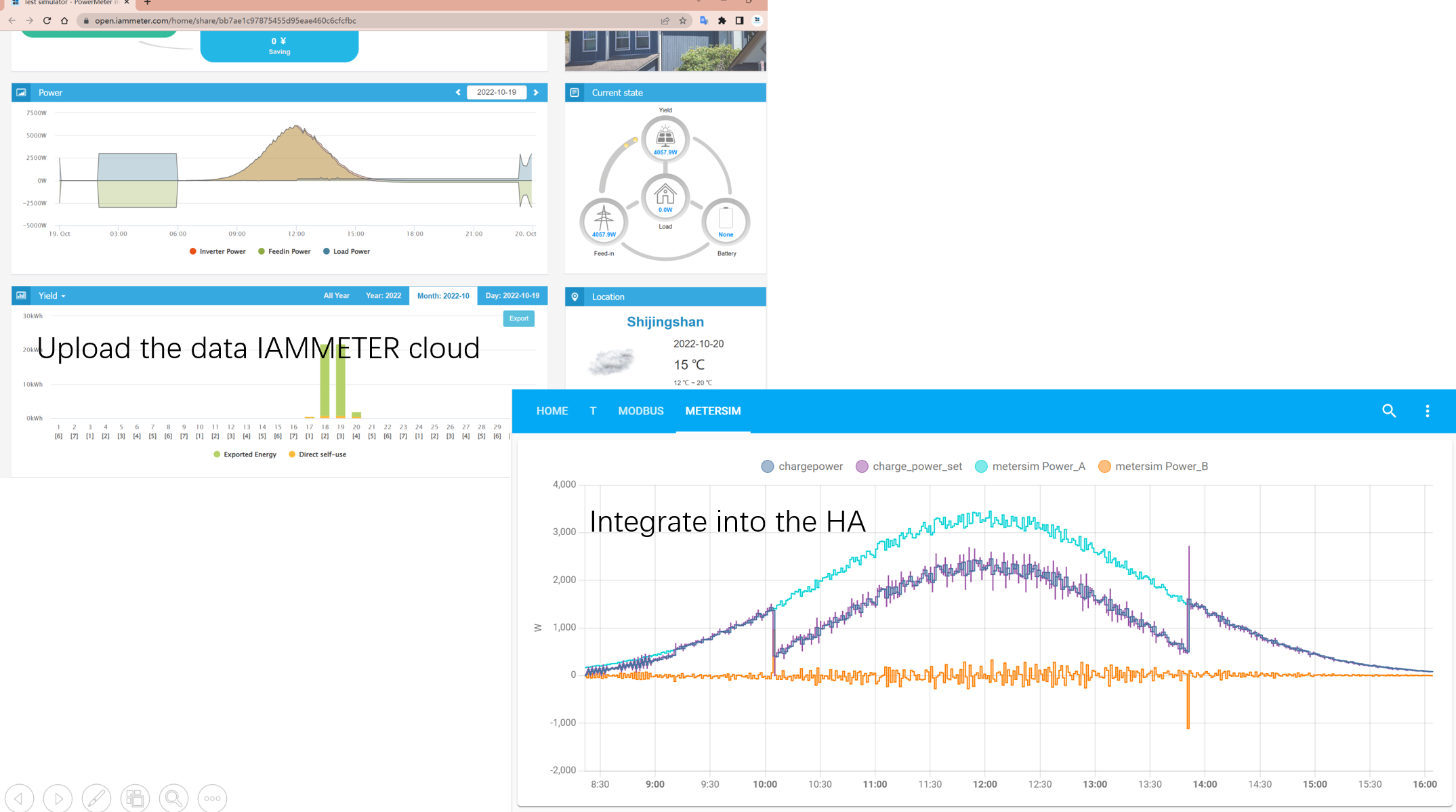# How it works

The simulator is developed by "ASP.NET Core", it is open source .

IAMMETER-simulator repository

After you had run this code,it assumes you had installed a 3-phase energy meter (WEM3080T) in this way.The simulator also supports the API of "monitorjson" like the real 3 phase energy meter.

The data of phase A in the simulator can come from totally simulated data(you can set the maximum power for the simulated inverter ), a real energy meter(WEM3080T), or the API return value from some platform(such as PVoutput).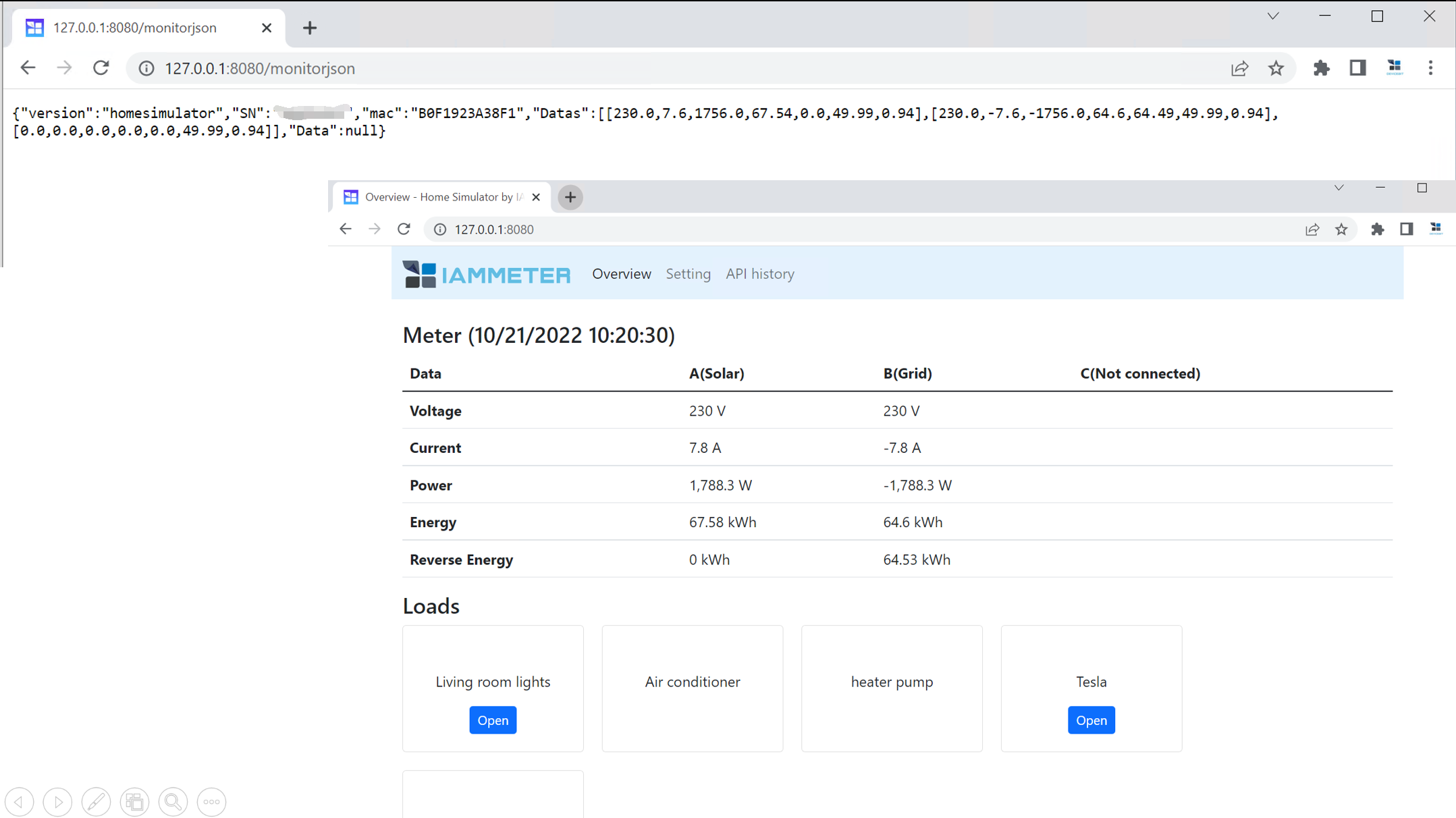# The load model in this simulator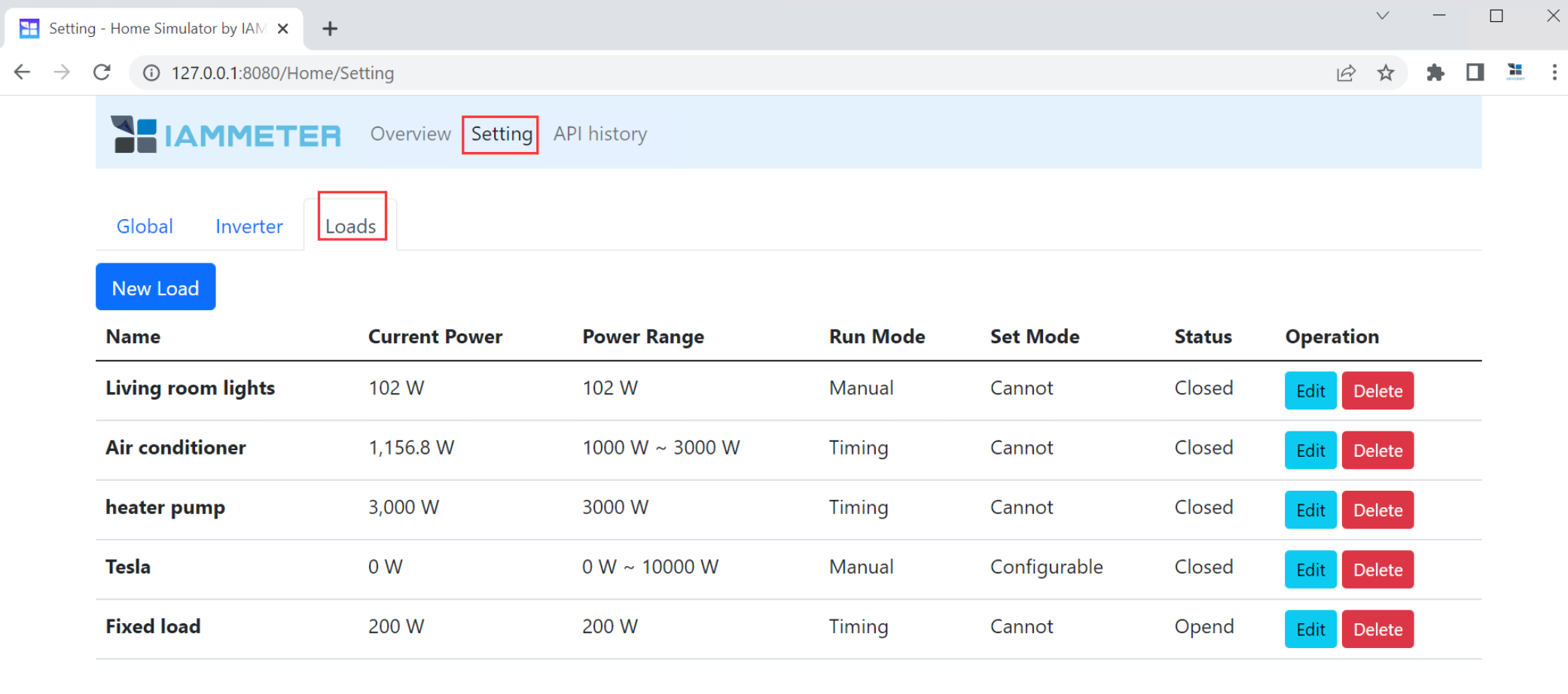The load model is used to simulate the real power consumption, there are three different load models which is supported now.

• Load with fixed power value that can be switched manually.
• Load value within a specific power range, run in a fixed time range.
• Load that can be controlled by an API (on/off ,or the specific power output like the tesla charger).

You can introduce your own control logic in this simulator to control such load models. Such as making some large load work in the time of off-peak tariff, using the solar excess output as more as possible.

Of course, it is not an easy way to provide a load model that can simulate the real situation. After we open source this code, we hope everyone interested in this project can improve the load model together, to make it closer to the real situation.

# Usage1：use the totally simulated data in this simulator

In this usage, all data is simulated by this simulator. Both solar PV output and load profile are simulated with regard to your setting.

The simulator will simulate the PV output and output its data in phase A. It will output a simulated load profile. It will calculate the grid output by the simulated PV data in phase A and the simulated load profile and output it in phase B.

## Simulate the solar PV output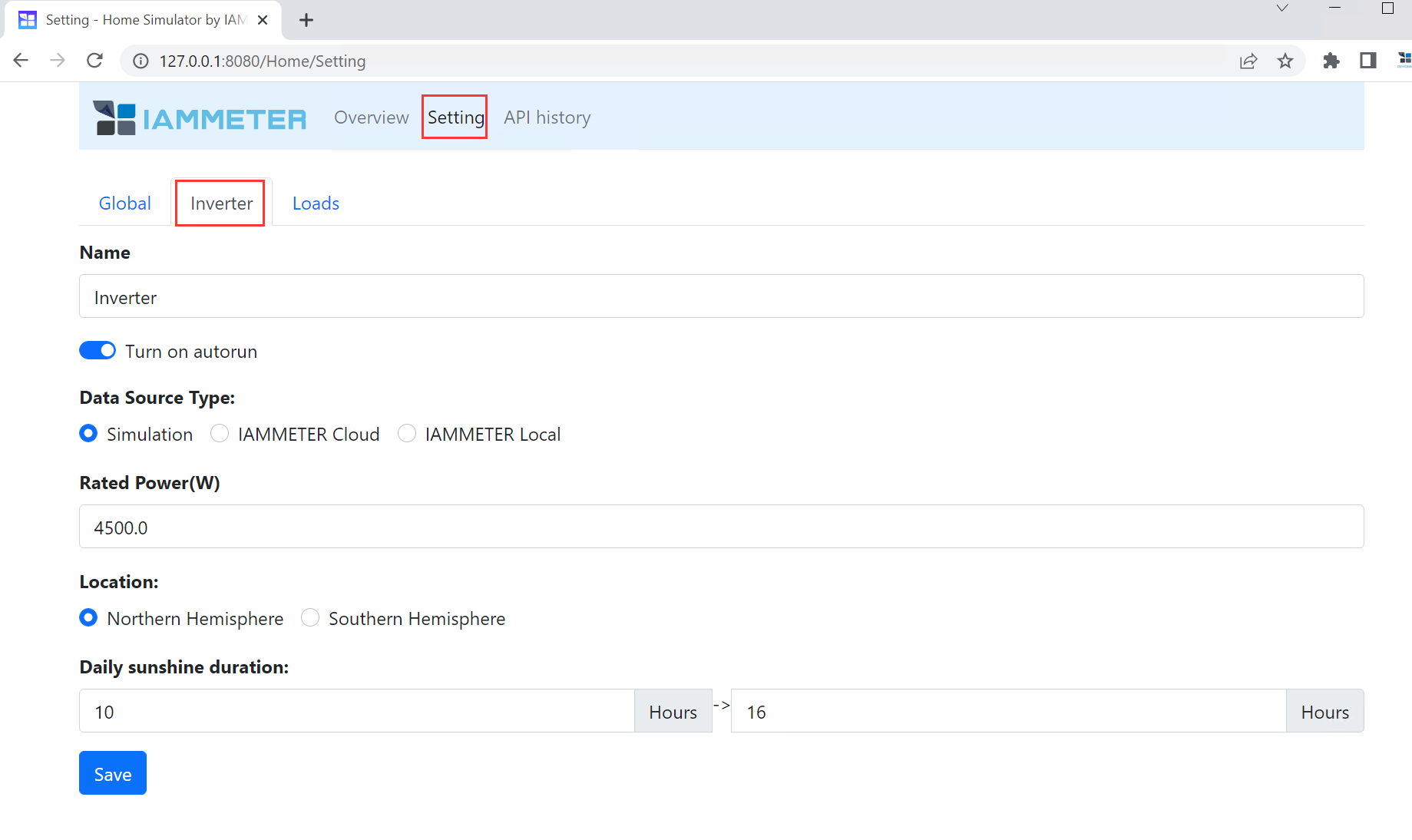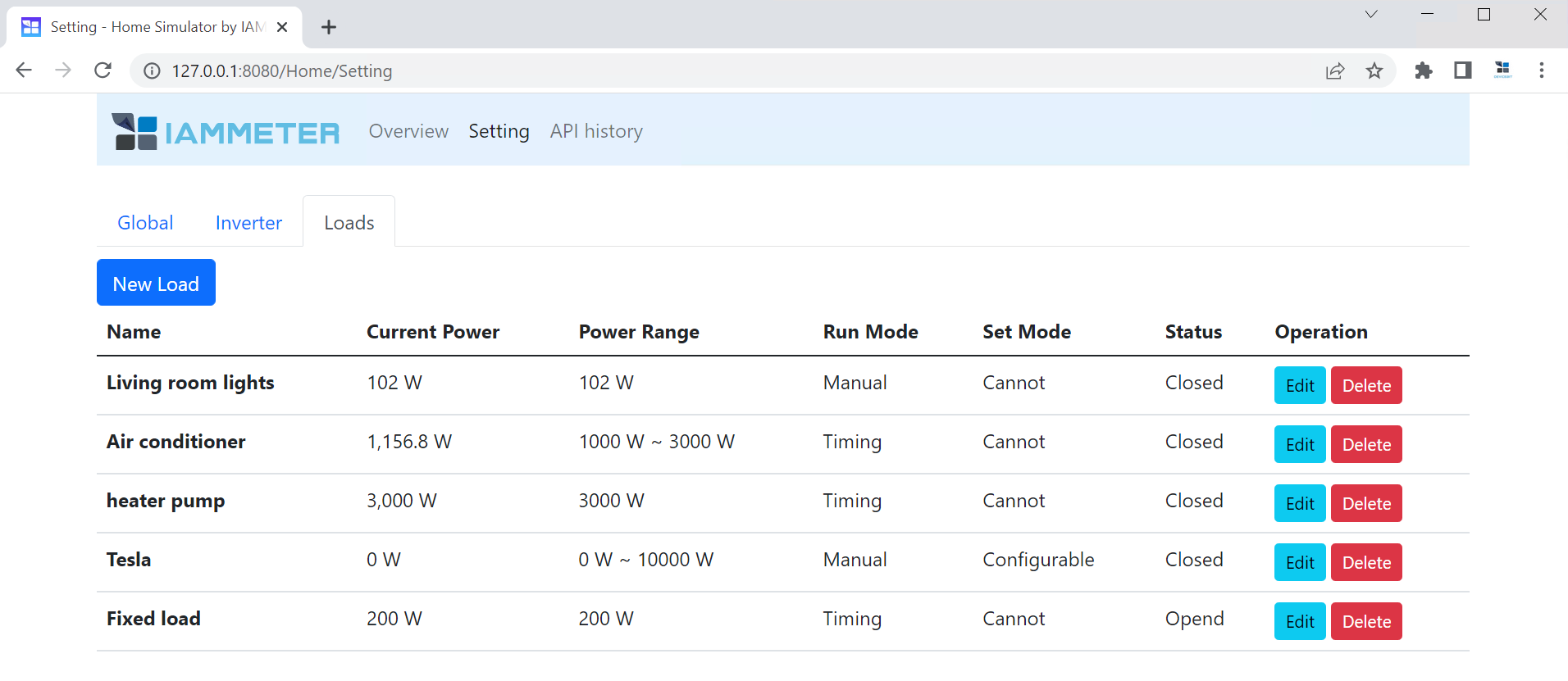## Read the data from the simulator and integrate it into Home Assistant

You can use get the data from the simulator by the same API ("monitorjson") that the real energy meter supports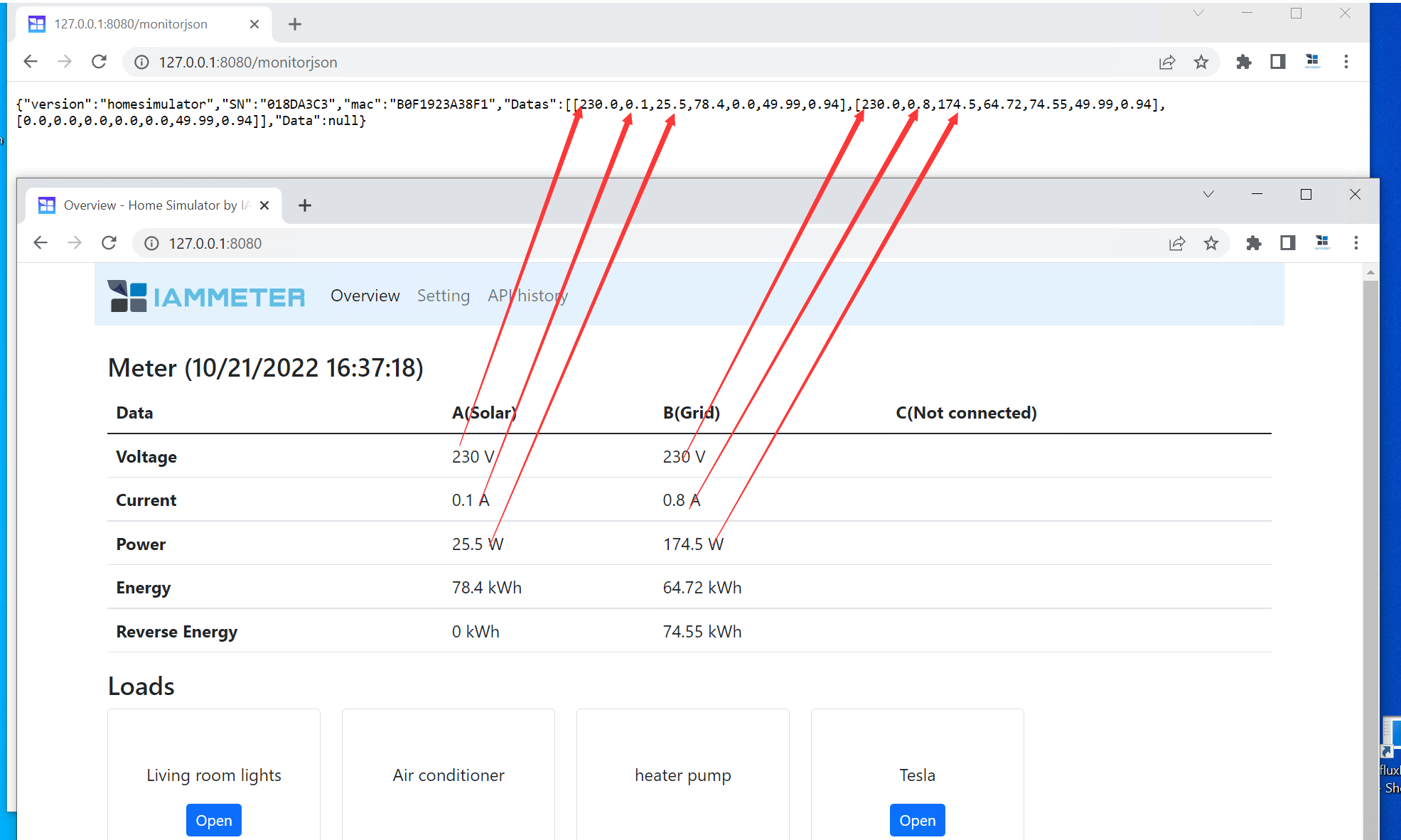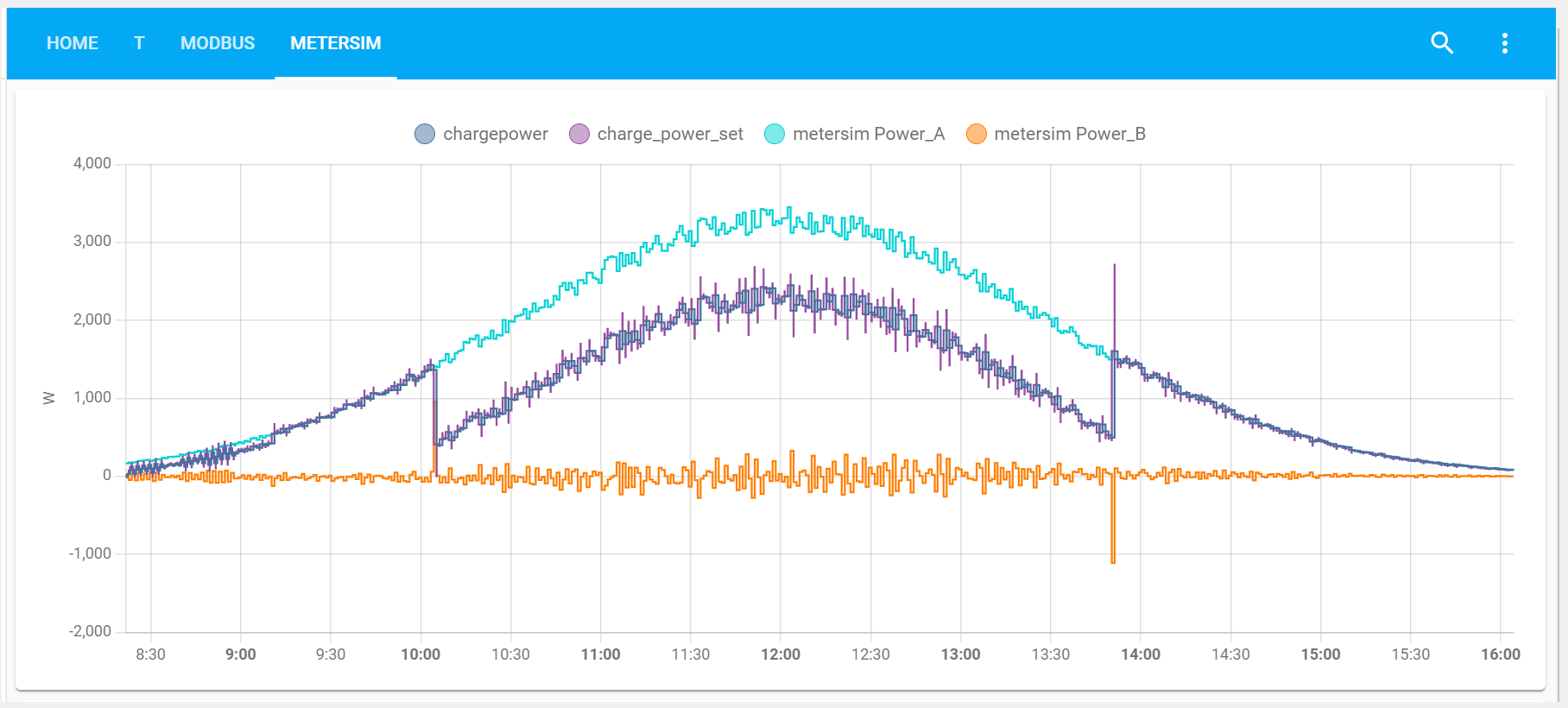It seems no sense to integrate a simulated energy meter into the Home assistant, but it will help you to understand this simulator well, then you would use the simulator better in usage 2 and usage 3.

# Usage 2: use the real solar PV output, find out the better control logic of your load

## Select the data source of the phase A in simulator

Now ,it only supports two interface, read from a real WEM3080T or read from the API of IAMMETER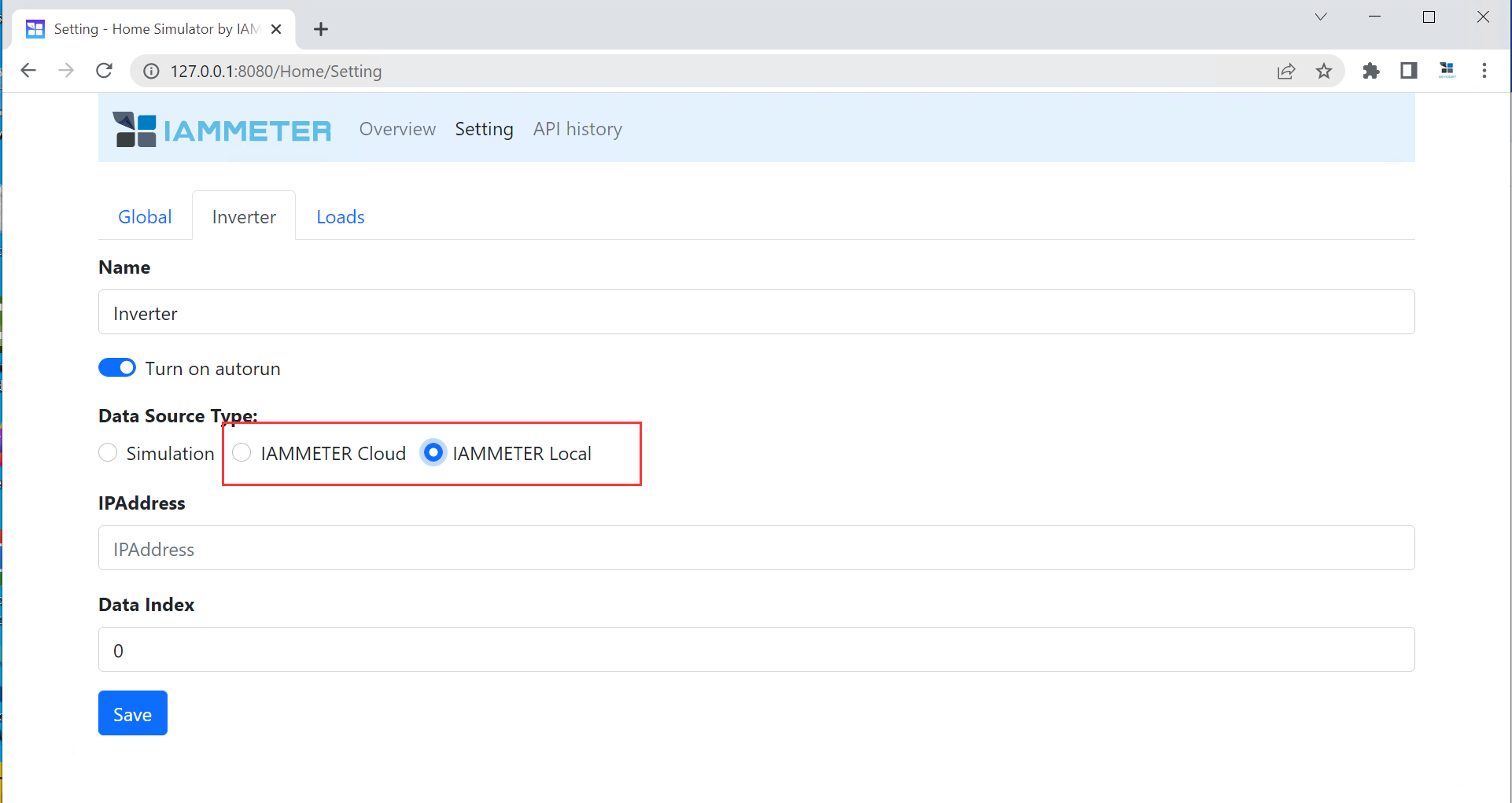In fact, any interface containing solar output data (whether read from real meters or get from online APIs) can be considered as a data source and added them here.

If you are familiar with other interfaces, welcome to submit PR to our repository.

Try to configure a load profile in the simulator, as close as your real situation.

Then the simulator will compute the grid consumption, with regard to this formula Grid power =solar output power - load power It will also compute the grid kWh data(both imported energy and exported energy) accordingly.

The simulator will output the grid data result (compute by the real solar and simulated load) in phase B .

You can read this simulated grid result by the local API "monitorjson", and integrate it into any platform you are familiar with.

• Power the load with the excess solar as much as possible.

• If the load can not power by solar(at night), then try to power it at the off-peak time as much as possible.

## Integrate the simulator into Home assistant to test your control logic

How to create a load profile and integrate it into the home assistant

# Ref

Run this simulator by the source code or Docker

How to create a load profile and integrate it into the home assistant

Integrate a virtual 3-phase energy meter (open source) into HA,use it to optimize your solar pv system

How to use IAMMETER`s Wi-Fi energy meter in the Home Assistant

Top